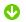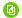## 二年级数学下册期末试卷 苏教版二年级下册数学期末试卷(一)

苏教版二年级下册数学期末试卷（一）

内容摘要：

1、直接写出得数。

50+60=         480－80=      600+900=     780－30=      50+600=

700－500=      72÷8=        6×9=        80+30=        40×2=

5×30=         20×7=        43×2=       2×13=        21×3=

...

 请下载附件： 1、苏教版二年级下册数学期末试卷（一） （共4页，本地下载在线阅读New！）

## 选择城市

#### 轻轻助手